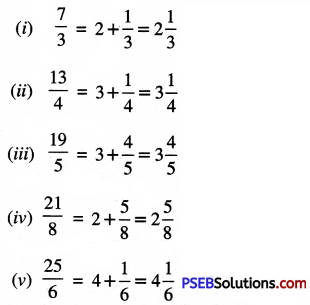# PSEB 6th Class Maths Solutions Chapter 5 Fractions Ex 5.2

Punjab State Board PSEB 6th Class Maths Book Solutions Chapter 5 Fractions Ex 5.2 Textbook Exercise Questions and Answers.

## PSEB Solutions for Class 6 Maths Chapter 5 Fractions Ex 5.2

1. Classify the following as proper and improper fractions:

Question (i)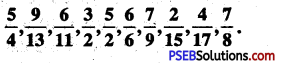Solution: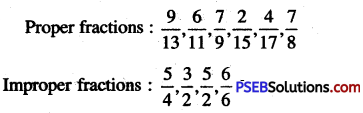2. Express each of the following as mixed fractions, Also represent with diagrams:

Question (i)
$$\frac {27}{5}$$
Solution: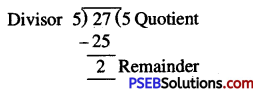∴ Mixed fraction
= Quotient $$\frac {Remainder}{Divisor}$$ = $$5\frac {2}{5}$$

Question (ii)
$$\frac {13}{4}$$
Solution: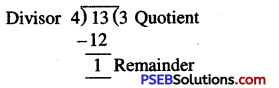∴ Mixed fraction
= Quotient $$\frac {Remainder}{Divisor}$$ = $$3\frac {1}{4}$$

Question (iii)
$$\frac {43}{8}$$
Solution: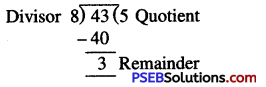∴ Mixed fraction
= Quotient $$\frac {Remainder}{Divisor}$$ = $$5\frac {3}{8}$$

Question (iv)
$$\frac {51}{7}$$
Solution: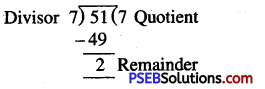∴ Mixed fraction
= Quotient $$\frac {Remainder}{Divisor}$$ = $$7\frac {2}{7}$$Question (v)
$$\frac {20}{3}$$
Solution: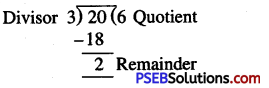∴ Mixed fraction
= Quotient $$\frac {Remainder}{Divisor}$$ = $$6\frac {2}{3}$$

3. Express each of the following mixed fractions as improper fractions:

Question (i)
(i) 2 \frac{1}{3}
(ii) 5 \frac{2}{7}
(iii) 4 \frac{3}{5}
(iv) 3 \frac{3}{4}
(v) 9 \frac{5}{8}
Solution: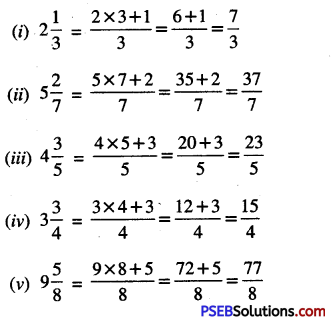4. Express the shaded portion as Improper Fraction and Mixed fraction:

Question (i)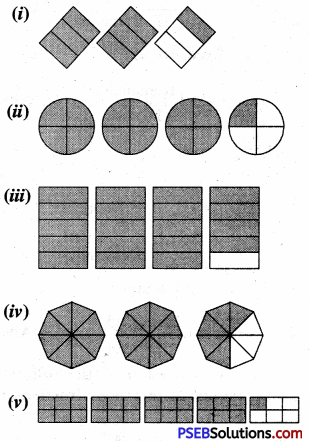Solution: